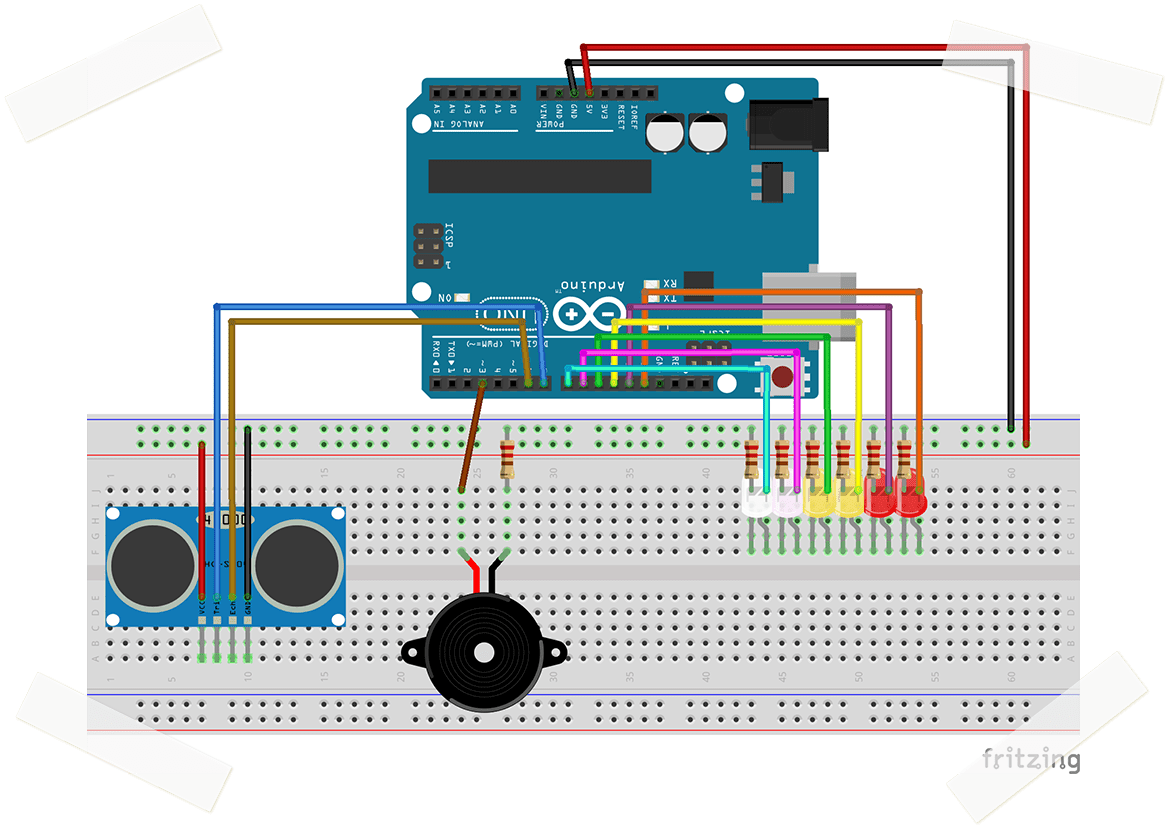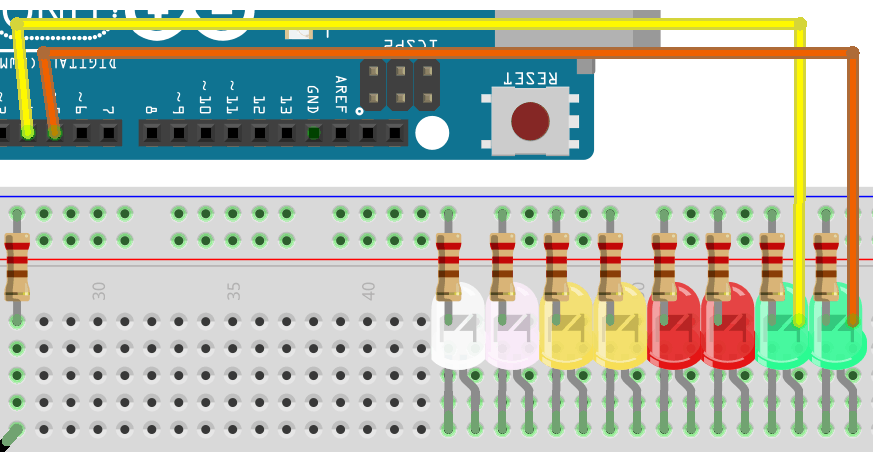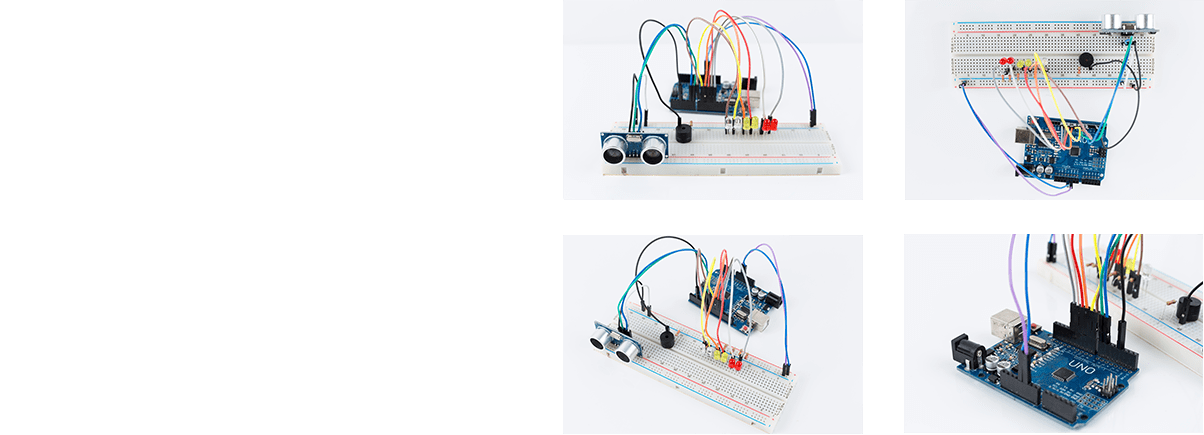# DISTANCE DETECTOR# EXERCISE SOLUTIONS:

Question 1

Most Ultrasonic Sensors can sense distances up to 400-500 cms. You will have to change the numbers(#) in these two sections of code:

if (distance <= #) { digitalWrite(white1, HIGH); sound = 900; }

if (distance > # || distance <= 0){ noTone(buzzer); }

Question 2

Replace this code:

if (distance > 40 || distance <= 0){ noTone(buzzer); }

With this code:

if (distance >= 10 || distance <= 0){ noTone(buzzer); }

Question 3

Change the order of the 'if statements':

if (distance < X)

The numbers (X) are currently 40, 30, 20, 15, 10, 5.
Reverse the order: 5, 10, 15, 20, 30, 40.

Bonus QuestionAdd the following lines of code in their appropriate sections:

#define green1 4
#define green2 5

pinMode(green1, OUTPUT); pinMode(green2, OUTPUT);

if (distance < 10) { digitalWrite(green1, HIGH); sound = 1300; }
else { digitalWrite(green1,LOW); }
if (distance < 5) { digitalWrite(green2, HIGH); sound = 1400; }
else { digitalWrite(green2,LOW); }